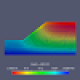# See the Convergence

Surely you have seen norms fly across the screen when running OpenSees with the print flag of the convergence test set to 1. The screen output slows down your analysis significantly, so you should only use print flag equal to 1 when you are trying to diagnose convergence issues.From a Jupyter Notebook. With OpenSees.exe, you’ll see all this in a DOS window.

Unless you redirect to a file and enjoy writing parsers built on a house of cards, the screen output is not very useful for plotting convergence behavior. Sure, you can spot check a few time steps to see if convergence is quadratic or linear. But that gets old really fast. There must be a better way.

A display recorder is available for viewing the residual and displacement increment vectors in real time during an analysis. I haven’t used this recorder in a long time, but as I recall, it’s good for seeing if the response at a DOF causes convergence problems, but not which DOF because the equations are typically renumbered.

Two little known utility functions, testNorm and testIter, make it possible to plot convergence from your script. Both functions operate on the most recent time step: testNorm returns a list of the norms at each iteration while testIter returns the number of iterations required for convergence.

Norms = []
for i in range(Nsteps):
ok = ops.analyze(1)

norms = ops.testNorm()
iters = ops.testIter()
for j in range(iters):
Norms.append(norms[j])

if ok < 0:
break

plt.semilogy(Norms,'k-x')

Note that the testNorm function returns a list with maxIter entries, where maxIter is the maximum number of iterations specified for the convergence test. Thus, the inner loop where values are appended based on the number of iterations returned by testIter. Yeah, I could have built a list of lists then flattened the result, but why be all Pythonic and import a bunch of stuff when a simple loop will do? Don’t tell me “for efficiency”, especially if you use Concrete23.

Anyway, I’ll demonstrate the above code with the simple two DOF spring system that I beat to death for a few weeks in my nonlinear structural analysis course.

Each spring uses a smooth arctangent function for its force-deformation response:

1.$\sigma=10\arctan(\varepsilon)$
2.$\sigma=4\arctan(0.5\varepsilon)$
3.$\sigma=7\arctan(\varepsilon)$

The load-displacement response of the spring system is computed using 12 steps of load control (Pref=1,$\Delta\lambda$=1).

Below are the norms of the residual vector for the two DOF spring analysis using Newton-Raphson and two variants–Modified Newton and Initial Newton. The convergence tolerance on the residual norm is 10-8.

As expected, Newton-Raphson converges quadratically while Modified Newton and Initial Newton converge linearly with Initial Newton converging more slowly.

You can do the same analysis with other algorithms, but the results aren’t all that interesting because the spring model has only two DOFs. In addition, the convergence rates won’t look as “textbook” for the multi-linear (non-smooth) force-deformation relationships we typically use for structural models.

## 2 thoughts on “See the Convergence”

1.Sukumar Baishya says:

Thanks Prof Scott for all your useful posts. They are equally enlightening for beginners as well as intermediate users like me.
Regards.

Liked by 3 people

1.bijanseif says:

I’m sure Also for professions. Thanks

Liked by 1 person

This site uses Akismet to reduce spam. Learn how your comment data is processed.